# How to divide a high-sense circle by mathematics into three equal parts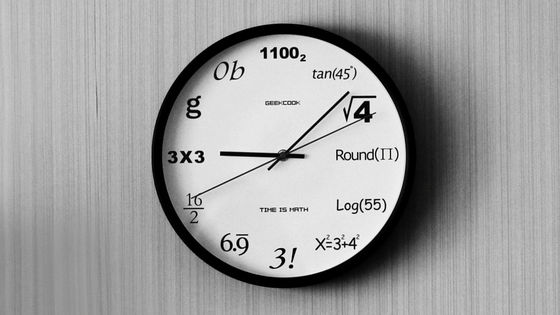by

wu yi

When cutting round foods such as whole cakes and pizzas into odd numbers such as three equal parts, it is often difficult to cut evenly, so it is often a big piece. There is even an app to solve such a difficult problem, but a group of people who love mathematics, “Meeting for Mathematics,” is recruiting and introducing cutting methods that are too high sense using mathematics.

Math love society (@mathlava) | Twitter

The beginning of the thing is the following tweet that the Twitter account of the society that loves mathematics tweeted.

The result is as follows. Five winners, one award for excellence, and one award for excellence were selected from the method of dividing the high-sense circle received from Twitter users who love mathematics into three equal parts.

“Concentric circle cut” seems to be divided into three equal parts by cutting the circle into four equal parts, then cutting them into concentric circles and dividing them into colored parts. The “pizza theorem” is a method that uses the pizza theorem . “Fruits are studded with fruits” is a method of cutting the lined parts cleanly.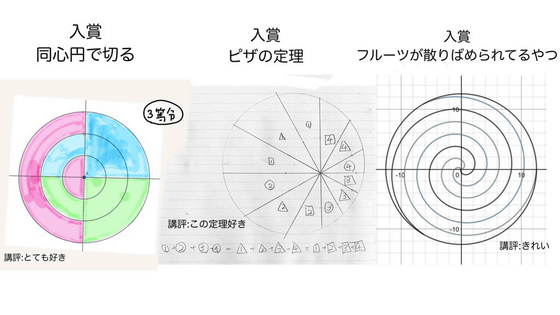“Cut by imagining a quadrant” is a method of cutting a circle into three equal parts while imagining a quadrant. It is the only practical work among the prize-winning works because it can be seen from which position the knife can be equally divided into three equal parts. “√3: √6: √9” means that the radius of the circle is cut concentrically at a ratio of √3: √6: √9.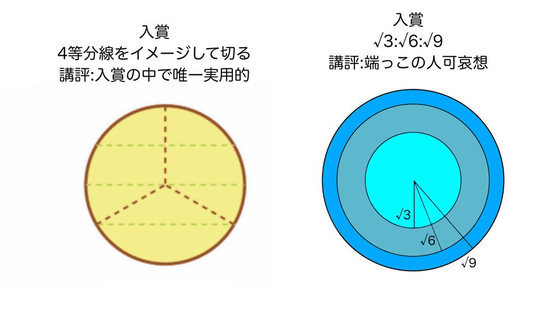The award for excellence is “Continuously divide into 4 equal parts”. The commentary says that 'you can't divide into 3 equal parts no matter how many years you cut' because it will continue to divide into 4 infinitely.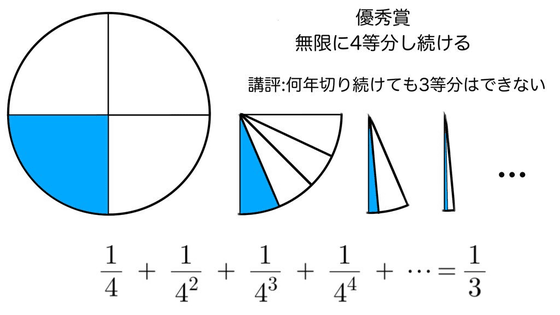The best award is 'cut with six-pointed stars'. If it is divided into colored parts, it will be divided into three equal parts.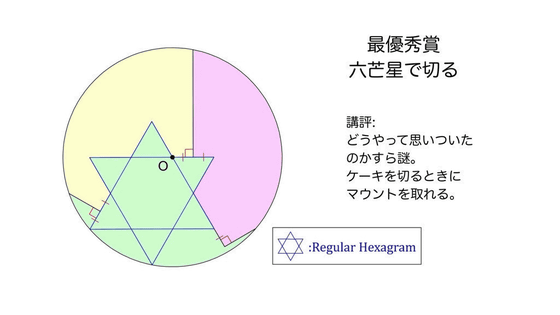When I calculated 'Is it really divided into three parts?'

The following image is a line drawn so that it can be seen clearly that the circle is divided into three equal parts with six-pointed stars. You can see that equilateral triangles of the same size are spread in the circle.

Ichiro Potato devised the best award.

From the following tweets, the reason why the mathematics-loving society suddenly solicited a method to divide the high-sense circle into three equal parts seems to be in the delinquent boys who do not cut cake .

In the past, mathematicians have invented a pizza cutting method that allows everyone to eat pieces that are easy to eat equally.

Invented by a mathematician `` How to cut pizza evenly and easily ''-GIGAZINE

in Note, Posted by logu_ii